# Preliminary Analysis of Gender Participation in AAS Talks¶

Initial analysis led by Brett Morris and Jim Davenport.

In :
%pylab inline
from urllib.request import urlopen
import datetime
import numpy as np
import matplotlib
matplotlib.rcParams['font.size'] = 15
from matplotlib import pyplot as plt

num = []
time = []
sid = []
speaker = []
qs = []

for i in range(len(raw)):
splitline = str(raw[i]).split(';').split(',')
splitline = [j for j in splitline if j != ' ' and j != '']

# If all columns filled and there is only one entry (M, F) for speaker gender:
if len(splitline) >= 5 and len(splitline) == 1 and ('M' in splitline.upper() or 'F' in splitline.upper()):
iscleansid = splitline.replace('.', '').isdigit()
if iscleansid:
# Fix sid if necessary
if len(splitline) != 6:
splitsid = splitline.split('.')
splitline = '.'.join([splitsid, splitsid.zfill(2)])

# If this talk ID has not been added:
if splitline not in sid:
num.append(splitline)
time.append(splitline)
sid.append(splitline)
speaker.append(splitline.upper())
qs.append(splitline.upper())

if splitline in sid:
# Check if the number of questions in new entry is longer.
# If so, replace earlier entry with the longer one.
if len(qs[sid.index(splitline)]) < len(splitline.upper()):
replaceindex = sid.index(splitline)
num[replaceindex] = splitline
time[replaceindex] = splitline
sid[replaceindex] = splitline
speaker[replaceindex] = splitline.upper()
qs[replaceindex] = splitline.upper()

Populating the interactive namespace from numpy and matplotlib

WARNING: pylab import has clobbered these variables: ['axes']
%matplotlib prevents importing * from pylab and numpy


## Overall demographics¶

In :
def annotateformat(number):
if number < 1:
return '%.2f' % number
else:
return '%d' % number

def nicehist(axis, female, male, title):
axis.bar([0, 1], [female, male], color='k', width=0.5)
axis.set_xticklabels(['Female', 'Male'])
axis.set_xticks([0.25, 1.25])
axis.set_xlim([-0.2, 1.7])

axis.annotate(annotateformat(female), xy=(0.25, female), va='bottom', ha='center')
axis.annotate(annotateformat(male), xy=(1.25, male), va='bottom', ha='center')
axis.set_title(title)
axis.spines['right'].set_color('none')
axis.spines['top'].set_color('none')
axis.xaxis.set_ticks_position('bottom')
axis.yaxis.set_ticks_position('left')

Nfemalespeakers = len([i for i in speaker if i == 'F'])
Nmalespeakers = len([i for i in speaker if i == 'M'])

fig, ax = plt.subplots(1)
nicehist(ax, Nfemalespeakers, Nmalespeakers,
'Gender of Speakers ({0} Total)'.format(Nfemalespeakers+Nmalespeakers))
plt.show()

print('Male speakers/All speakers= {0:.1f}%'
.format(100*float(Nmalespeakers)/(Nfemalespeakers + Nmalespeakers)))
print('Female speakers/All speakers = {0:.1f}%'
.format(100*float(Nfemalespeakers)/(Nfemalespeakers + Nmalespeakers)))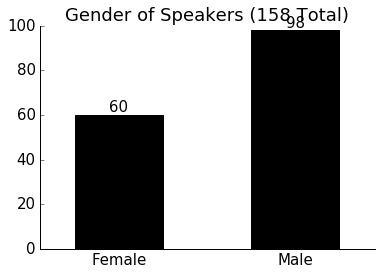Male speakers/All speakers= 62.0%
Female speakers/All speakers = 38.0%

In :
allqs = ''.join([i for i in qs if 'M' in i or 'F' in i])
Nfemaleqs = len([i for i in allqs if i == 'F'])
Nmaleqs = len([i for i in allqs if i == 'M'])

fig, ax = plt.subplots(1)
nicehist(ax, Nfemaleqs, Nmaleqs, 'Gender of Question-Askers (%d Total)' % (Nfemaleqs+Nmaleqs))

print('Male qs/All qs = {0:.1f}%'.format((100*float(Nmaleqs)/(Nfemaleqs + Nmaleqs))))
print('Female qs/All qs = {0:.1f}%'.format((100*float(Nfemaleqs)/(Nfemaleqs + Nmaleqs))))

Male qs/All qs = 81.1%
Female qs/All qs = 18.9%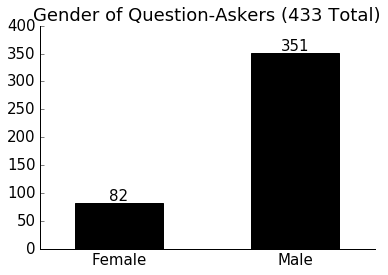In :
fig, ax = plt.subplots(1)
Nbins = 7

ax.hist([len(i) for i in qs], Nbins, histtype='stepfilled', color='w', lw=2)
ax.set_title('Number of Questions Per Talk:')
ax.set_xlabel('Number of Questions')
plt.show()

malespeaker_Nqs = [len(qs[i]) for i in range(len(num)) if speaker[i] == 'M']
femalespeaker_Nqs = [len(qs[i]) for i in range(len(num)) if speaker[i] == 'F']

fig, ax = plt.subplots(1)
ax.hist(malespeaker_Nqs, Nbins, histtype='step', color='b', lw=2, label='Male Speaker', range=[1,8])
ax.hist(femalespeaker_Nqs, Nbins, histtype='step', color='r', lw=2, label='Female Speaker', range=[1,8])
ax.set_title('Number of Questions Per Talk:')
ax.set_xlabel('Number of Questions')
ax.legend()
plt.show()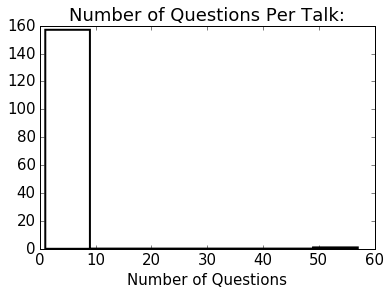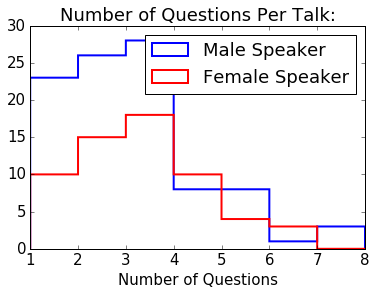In :
Nmalesfirst = len([i for i in qs if i == 'M'])
Nfemalesfirst = len([i for i in qs if i == 'F'])

fig, ax = plt.subplots(1)
nicehist(ax, Nfemalesfirst, Nmalesfirst,
'Gender of First Question-Askers ({0} Total)'.format(Nfemalesfirst+Nmalesfirst))
plt.show()

print('Male first qs/All qs = {0:.1f}%'.format((100*float(Nmalesfirst)/(Nfemalesfirst + Nmalesfirst))))
print('Female first qs/All qs = {0:.1f}%'.format((100*float(Nfemalesfirst)/(Nfemalesfirst + Nmalesfirst))))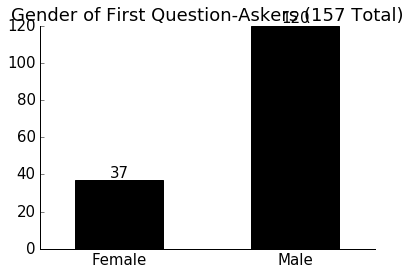Male first qs/All qs = 76.4%
Female first qs/All qs = 23.6%


## When males/females ask first Q, are subsequent Qs affected?¶

In :
malesfirst = [i for i in qs if i == 'M']
malesfirst_percentagefemaleafter = [float(i.count('F'))/len(i) for i in malesfirst[1:] \
if len(i) >= 2]

femalesfirst = [i for i in qs if i == 'F']
femalesfirst_percentagefemaleafter = [float(i.count('F'))/len(i) for i in femalesfirst[1:]
if len(i) >= 2]

fig, ax = plt.subplots(1, 2, figsize=(16,8))
Nbins = 5

ax.hist(malesfirst_percentagefemaleafter, Nbins, color='k',
range=[0,1], histtype='step', lw=2,
weights=len(malesfirst_percentagefemaleafter)*[1./len(malesfirst_percentagefemaleafter)])

ax.hist(femalesfirst_percentagefemaleafter, Nbins, color='k',
range=[0,1], histtype='step', lw=2,
weights=len(femalesfirst_percentagefemaleafter)*[1./len(femalesfirst_percentagefemaleafter)])

for axes in ax:
axes.set_xlim([0, 1])
axes.set_xlabel("Fraction subsequent Q's asked by females")
axes.set_ylabel("Fraction of all talks")
axes.set_ylim([0, 1])
plt.show()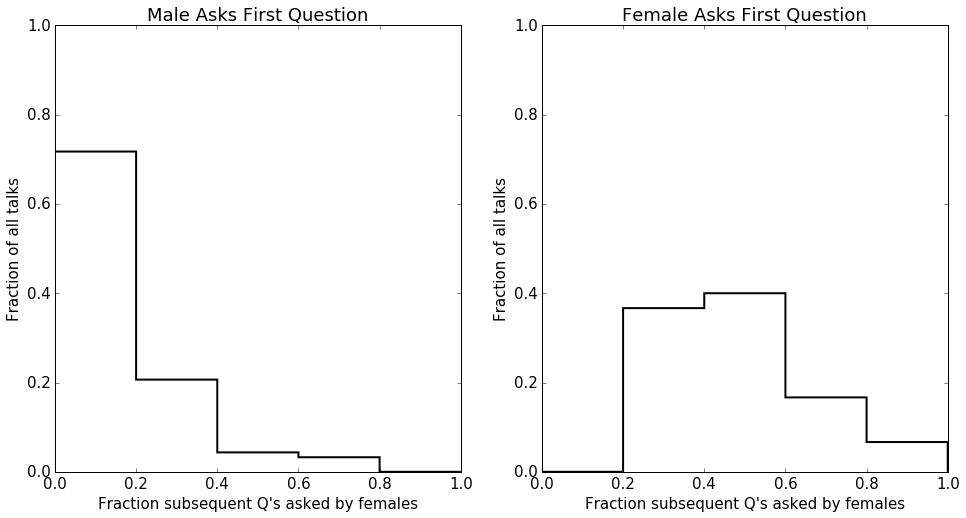In :
fig, ax = plt.subplots(1, figsize=(10,8))
Nbins = 5
ax.hist(malesfirst_percentagefemaleafter, Nbins, color='b',
range=[0,1], histtype='step', lw=3,
weights=len(malesfirst_percentagefemaleafter)*[1./len(malesfirst_percentagefemaleafter)],

ax.hist(femalesfirst_percentagefemaleafter, Nbins, color='r',
range=[0,1], histtype='step', lw=3,
weights=len(femalesfirst_percentagefemaleafter)*[1./len(femalesfirst_percentagefemaleafter)],

ax.set_xlim([0, 1])
ax.set_ylim([0, 1])
ax.set_xlabel("Fraction subsequent Q's asked by females")
ax.set_ylabel("Fraction of all talks")
ax.spines['right'].set_color('none')
ax.spines['top'].set_color('none')
ax.xaxis.set_ticks_position('bottom')
ax.yaxis.set_ticks_position('left')
ax.legend()
plt.show()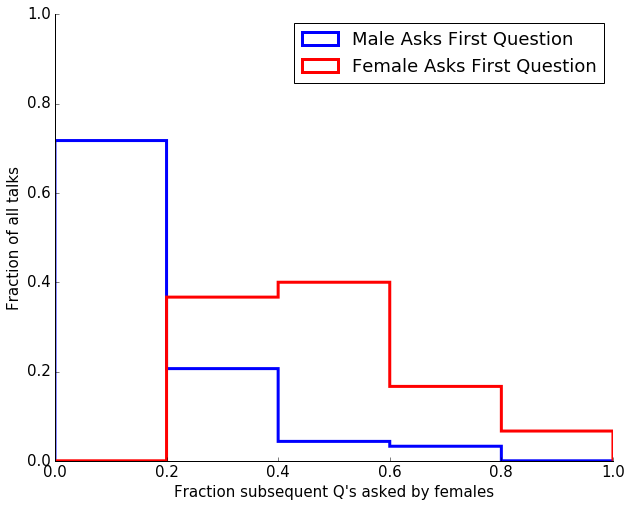In :
malesfirst_lenafter = float(len(''.join(malesfirst)))
malesfirst_Nfemaleafter = sum([i.count('F') for i in malesfirst[1:]
if len(i) >= 2])/malesfirst_lenafter
femalesfirst_lenafter = float(len(''.join(femalesfirst)))
femalesfirst_Nfemaleafter = sum([i.count('F') for i in femalesfirst[1:]
if len(i) >= 2])/femalesfirst_lenafter

fig, ax = plt.subplots(1, 2, figsize=(16,8))

nicehist(ax, malesfirst_Nfemaleafter, 1-malesfirst_Nfemaleafter,
'Male Asks First Q ({0} Total)'.format(len(''.join(malesfirst))))

nicehist(ax, femalesfirst_Nfemaleafter, 1-femalesfirst_Nfemaleafter,
'Female Asks First Q (%d Total)'.format(len(''.join(femalesfirst))))

for axes in ax:
axes.set_ylim([0,1])

plt.show()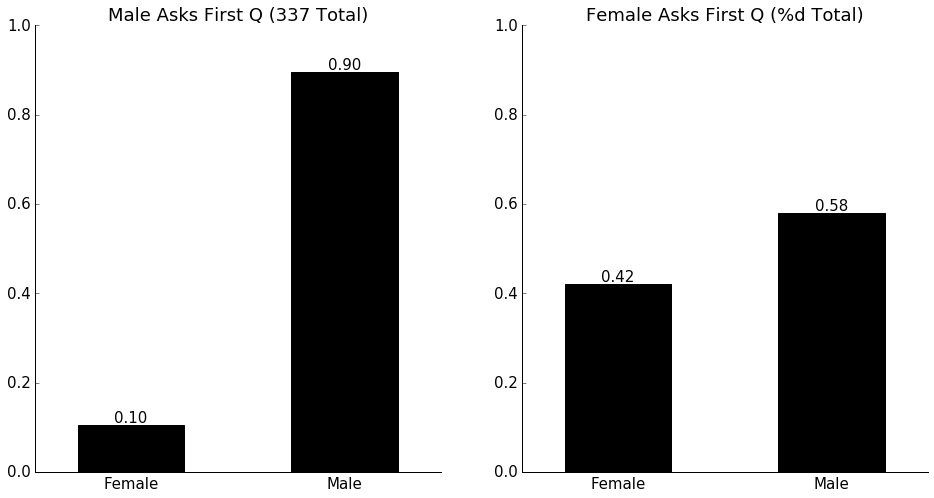In :
malespeaker_qs = [qs[i] for i in range(len(num)) if speaker[i] == 'M']
femalespeaker_qs = [qs[i] for i in range(len(num)) if speaker[i] == 'F']

malespeaker_Nfemaleqs = ''.join(malespeaker_qs).count('F')
malespeaker_Nquestions = len(''.join(malespeaker_qs))
malespeaker_percentagefemaleqs = malespeaker_Nfemaleqs/float(malespeaker_Nquestions)

femalespeaker_Nfemaleqs = ''.join(femalespeaker_qs).count('F')
femalespeaker_Nquestions = len(''.join(femalespeaker_qs))
femalespeaker_percentagefemaleqs = femalespeaker_Nfemaleqs/float(femalespeaker_Nquestions)

fig, ax = plt.subplots(1, 2, figsize=(16,8))

nicehist(ax, malespeaker_percentagefemaleqs, 1-malespeaker_percentagefemaleqs,
'Male Speaker ({0} Total)'.format(malespeaker_Nquestions))

nicehist(ax, femalespeaker_percentagefemaleqs, 1-femalespeaker_percentagefemaleqs,
'Female Speaker ({0} Total)'.format(femalespeaker_Nquestions))
for axes in ax:
axes.set_ylim([0,1])

plt.show()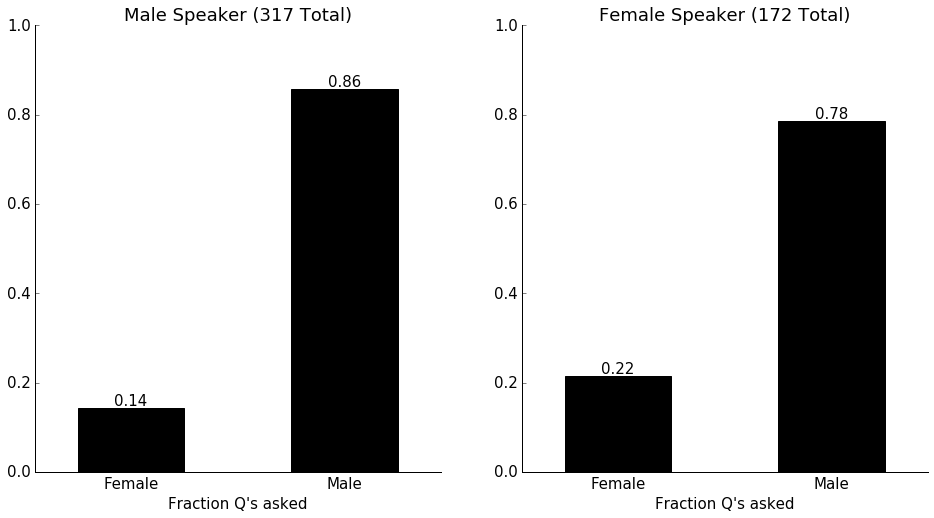## When is first question asked by female?¶

In :
def getfirstindex(searchstring, letter):
if letter in searchstring:
return searchstring.index(letter) + 1
return None

malespeaker_orderfemale = [getfirstindex(i, "F") for i in malespeaker_qs if 'F' in i]
femalespeaker_orderfemale = [getfirstindex(i, "F") for i in femalespeaker_qs if 'F' in i]

fig, ax = plt.subplots(1, figsize=(10,8))
Nbins = 3
ax.hist(malespeaker_orderfemale, Nbins, color='b',
range=[1,3], histtype='step', lw=3,
label='Male Speaker')

ax.hist(femalespeaker_orderfemale, Nbins, color='r',
range=[1,3], histtype='step', lw=3,
label='Female Speaker')

ax.set_xlim([1, 3])
ax.set_xticks([1,2,3])
#ax.set_ylim([0, 1])
ax.set_xlabel("First Female Question Position in Sequence")
#ax.set_ylabel("Fraction of all talks")
ax.set_title('In talks when a female asked a question:')
ax.spines['right'].set_color('none')
ax.spines['top'].set_color('none')
ax.xaxis.set_ticks_position('bottom')
ax.yaxis.set_ticks_position('left')
ax.legend()
plt.show()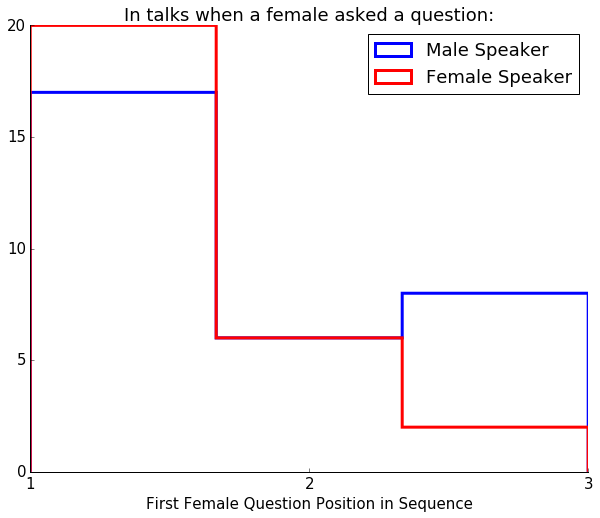In :
N_qs = np.array([len(i) for i in qs])
percentagefemaleqs = np.array([i.count('F')/float(len(i)) for i in qs])
order = np.argsort(N_qs)

#plt.plot(N_qs, percentagefemaleqs, '.')

for i in range(1, max(N_qs)):
considertalks = i == N_qs
medianpercentagefemaleqs = np.median(percentagefemaleqs[considertalks])
stdpercentagefemaleqs = np.std(percentagefemaleqs[considertalks])
plt.errorbar(i, medianpercentagefemaleqs, yerr=stdpercentagefemaleqs, fmt='o', color='k')
plt.ylim([0, 1.0])
plt.ylabel('Fraction of Qs asked by females')
plt.show()

/Users/bmmorris/anaconda/lib/python3.5/site-packages/numpy/core/_methods.py:59: RuntimeWarning: Mean of empty slice.
warnings.warn("Mean of empty slice.", RuntimeWarning)
/Users/bmmorris/anaconda/lib/python3.5/site-packages/numpy/core/_methods.py:70: RuntimeWarning: invalid value encountered in double_scalars
ret = ret.dtype.type(ret / rcount)
/Users/bmmorris/anaconda/lib/python3.5/site-packages/numpy/core/_methods.py:82: RuntimeWarning: Degrees of freedom <= 0 for slice
warnings.warn("Degrees of freedom <= 0 for slice", RuntimeWarning)
/Users/bmmorris/anaconda/lib/python3.5/site-packages/numpy/core/_methods.py:94: RuntimeWarning: invalid value encountered in true_divide
arrmean, rcount, out=arrmean, casting='unsafe', subok=False)
/Users/bmmorris/anaconda/lib/python3.5/site-packages/numpy/core/_methods.py:116: RuntimeWarning: invalid value encountered in double_scalars
ret = ret.dtype.type(ret / rcount)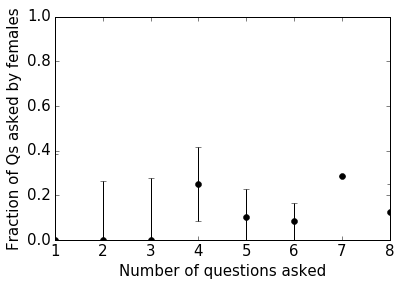## Correlations by genre¶

In :
infile = 'aas225-all-talks.txt'

rawchairgender = []
catagory = []
sessionID = []

for i,line in enumerate(raw):
if i % 5 == 3:
a, b, = line.split(',')
catagory.append(a)
rawchairgender.append(b.strip())
if i % 5 == 0:
sessionID.append(line[0:3])

rawchairgender = np.array(rawchairgender)
sessionID = np.array(sessionID)
chair = []
for talknumber in sid:
session = talknumber.split('.')
chair.append(rawchairgender[sessionID == session])

In [ ]:



# Questions to ask the data:¶

Show how reliable responses are by looking for simultaneous responses from individual talks?

Does the order of questions asked matter?

Are females more likely to ask follow-up questions rather than leading quetions?

Do women ask N questions proportionate to their size in the population?

If a woman asks a question, are others more likely to follow? Clustering?

Are the speaker genders similar in M/F to the overall population?

What are the biases in the talks that get survey responses?

Do people submit responses more often for talks given by women compared to the general pop?

How late in Q session is the first female Q?

In [ ]: